# Maths & stuff

A collection of various documents on how to do some trivial math stuff usually encountered in 3d graphics.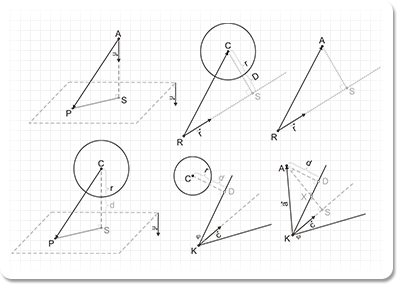A collection of some of the frequently occuring tasks.
Includes:
• Distance between a point and a plane
• Distance between a sphere and a plane
• Distance between ray and perpendicular plane
• Distance between a point and a ray
• Distance between a sphere and a ray
• Distance between point and a cone
• Distance between a sphere and a cone
• Angle between ray and plane

## Calculating barycentric coordinatesA doc on how to calculate barycentric coordinates. This method is used in the ray-triangle intersection calculation to produce the UV coordinates of the intersection.

## Closest point between two rays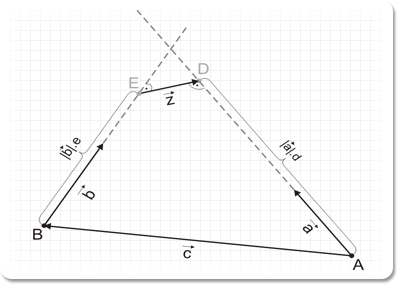This doc unrolls on how to find the closest point between two rays. Along the way, it also shows how to get the shortest section between the rays and how to determine if the rays are intersecting.

## Calculating the intersection point between a ray and a plane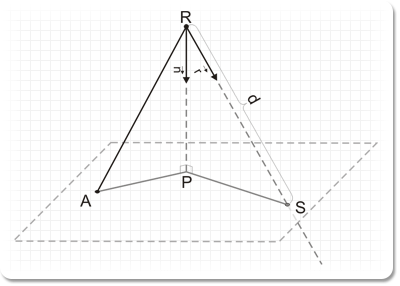Just as the name suggests, the document shows how to calculate where a ray intersects a point. This method is used in the ray-triangle intersection calculation.

## Calculating the intersection point between a ray and a triangle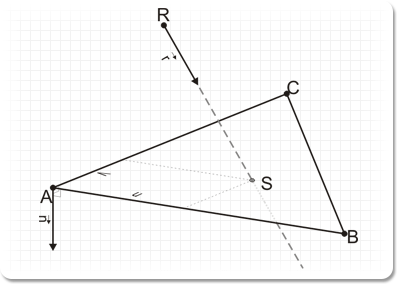This doc shows how to calculate if and where a ray intersects with a triangle. It also shows how to calculate the barycentric coordinates of the intersection. Everything a typical 3D mesh picking algorithm needs.

## Converting between camera lens and FOV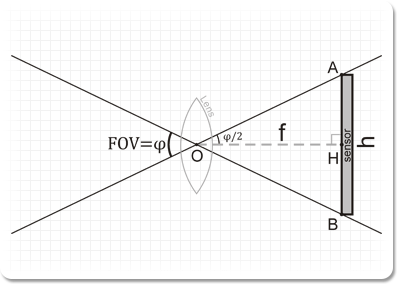Shows how to convert between a camera lens and its respective field of view. The method takes into consideration the camera's focal length and film size and gives a few words on how they fit in 3DS Max

## Calculating tangent vectors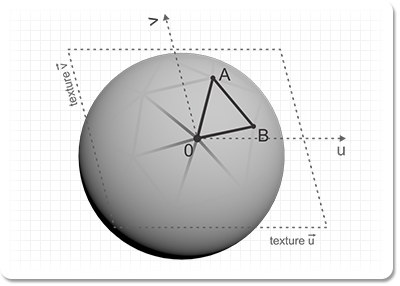This doc shows how to calculate the tangent and bitangent vectors (sometimes somewhat erroneously referred to as tangent and binormal) for a vertex of a polygon and briefs on how normal mapping is applied.